### Testing Linear Hypotheses about the Parameters

Linear hypotheses for parameters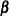are expressed in matrix form as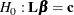whereis a matrix of coefficients for the linear hypotheses andis a vector of constants.

Suppose that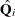and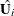are the point and covariance matrix estimates, respectively, for a p-dimensional parameter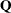from the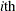imputed data set, i=1, 2, …, m. Then for a given matrix, the point and covariance matrix estimates for the linear functions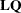in theimputed data set are, respectively,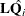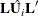The inferences described in the section Combining Inferences from Imputed Data Sets and the section Multivariate Inferences are applied to these linear estimates for testing the null hypothesis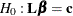.

For each TEST statement, the Test Specification table displays thematrix and thevector, the Variance Information table displays the between-imputation, within-imputation, and total variances for combining complete-data inferences, and the Parameter Estimates table displays a combined estimate and standard error for each linear component.

With the WCOV and BCOV options in the TEST statement, the procedure displays the within-imputation and between-imputation covariance matrices, respectively.

With the TCOV option, the procedure displays the total covariance matrix derived under the assumption that the population between-imputation and within-imputation covariance matrices are proportional to each other.

With the MULT option in the TEST statement, the Multivariate Inference table displays an F test for the null hypothesis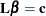of the linear components.